# Enoch’s Rod of π is the Revelation of Jesus##### Enoch and his calendar

(This represents my earliest work on Pi. While accurate, the final formula is explained at this link.)

The following formula is 1000 times more accurate than NASA uses for all its space endeavors. Yet it unfolds Enoch’s calendar (and all calendars) and is the numerical sum of much that is found in nature and the Bible.

As discussed in previous documents, (written before this formula was discovered)…

• 364 is a year on Enoch’s calendar
• 365 is a solar Year
• 25920 and 25800 years are the “Great Year“, that is, Precession of the Equinox. And as the 1/25920th part of a day (and thus 1/25800th, too), it is the smallest unit according to Babylonian and Rabinnic reckoning (= 3.33… seconds)

There are different ways to present the same formula. We begin with the simplest.

## The Rod of Enoch is Pi π!

Ponder the first term and Gen 1:14-16; 2:2Lev 23:39.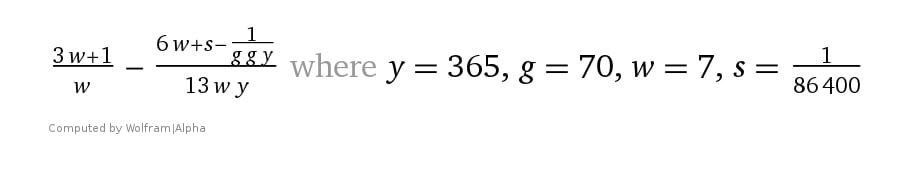y =year, g = generation, w = week, s = second. Each term is successively more accurate to π, reaching 14 decimals of pi. The last term can also be written as 100 jubilees, ie 4900 years. Notice that “y = 365, g = 70, w = 7” is Daniel’s “seventy weeks of years” from Daniel 9:24-27, whereas the similar “g g y” (i.e., 70 generations”) is the time allotted for the imprisonment of the angels as per the Book of Enoch, 10:12. Perhaps this is the greatest evidence given to modern man that God created all things. If you know someone with even a basic understanding of math, astronomy or Bible prophecy, please email the formula to them along with this brief explanation or put it on Facebook! 1260d.com

The below formula is the same as the above but instead of adding “one second” it adds 3½ “parts” instead. (A “part” is the smallest part of a day according to Jewish and Babylonian reckoning.)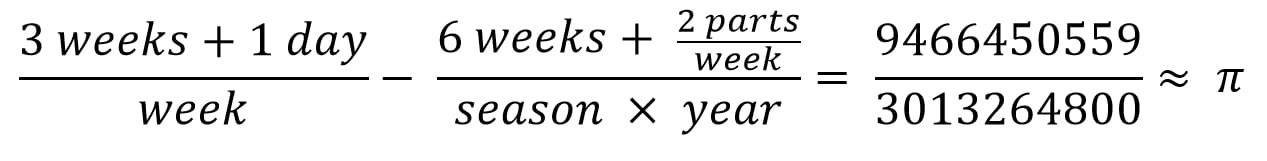“The Rod of Enoch”. Astonishingly, this formula is accurate to 12 decimals of Pi. It encodes every aspect of our calendar year, including seconds, “parts“, days, weeks, months, seasons, years, Sabbath years, jubilees, even the vast “Years” of Precession of 25920 years, etc. It also includes all the Jewish festivals of the Bible

The numerator (circumference) equals “≈ 1000 Years of Precession as if just one day, and a day as 1000 Years”, (2Pet 3:8).

##### Daniel’s “seventy weeks”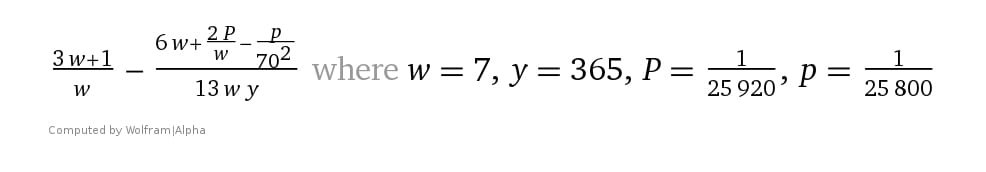This version contains the next logical term, accurate to Pi (π) to 18 decimals. In the Bible, “25800” years” is also Precession, (and is 1/60th of “430”). To be consistent, therefore, the day can also be broken down as 1/25800th of a day. Also, “70²” (i.e., 70 x 70) is the timeframe given in the Book of Enoch that the fallen angels are locked up in their prisons and is inferred to in “Daniel’s seventy weeks“, (Daniel 9:24-29).This is part of an ongoing series on the Calendar of Enoch.

The above formula is the subtle blueprint that underlies all biblical patterns and of time itself.

## Other methods of the same formula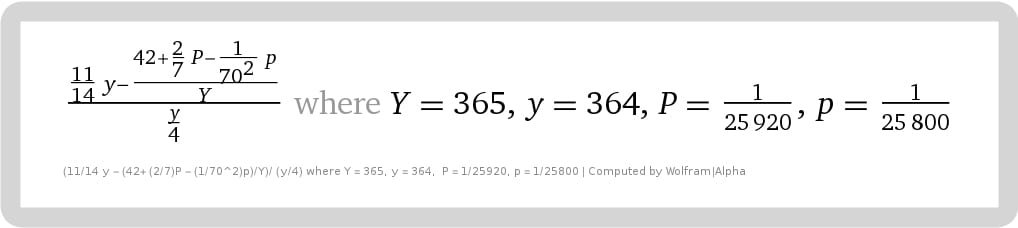Pi: The Rod of Enoch. This formula locates a position in space/time of a straight line surrounded by its circle, which by definition is π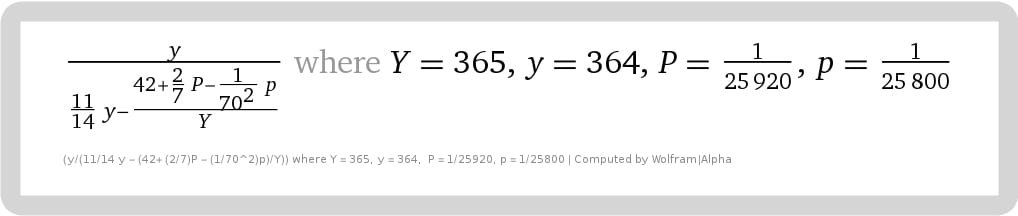Pi: The Rod of Enoch. The formula locates a position in space/time of the perimeter of the square to its corresponding circle, symbolic of earth and water respectively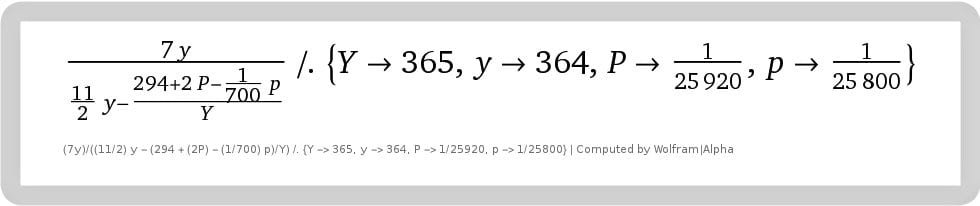Pi: The Rod of Enoch, here converted into units of seven (“weeks”) instead of “one” that reveal other relationships to the Enoch calendar. (Example, “the week” and “294” (7*7*6) are crucial cycles in the calendar. Moreover, keep in mind that a day is as a year (or “a 1000 years”) and a year is as a day”, (2Peter 3:8)

Below is the formula of the ratio of Perimeter to Circumference expressed as a fraction.

226748176200000/178087601141201

y/((11/14) y – (42 + (2/7) P – (1/70^2) p)/Y) /. {Y -> 365, y -> 364, P -> 1/25920, p -> 1/25800}

(Four divided into the above = π rationalized, i.e., 178087601141201/56687044050000.)

### The formula multiplied by 1 season of Enoch (91)An expanded version of the formula for Pi in space and time. Keep in mind that “1274” is simply 364*3.5, and is, therefore, the equivalent of 1260 days (Rev. 11). The 1000 years begins the last Sabbatical cycle of seven-year periods that “cuts short” 21 weeks, less “the twinkling of an eye” of the full 1001 years. In part, it has to do with the tithe (10%). Example, 441 + 49 = 490 (Dan 9); 7*365 less 10% = 2300 (Dan 5,8,12), and here, +/- 10% of 1000 (and 1oo1) x 365 (0r 360 or 364) (&*4), etc.

I will elaborate later, especially the rationalization of π using continual fractions, how that it unravels the above formula in stages.

## ‘The Rod of Pi’ Fractal

Fractals are formed by variations of circles -Pi.“The Rod of Enoch is Pi” (fractal). Click to enlarge to view the middle figure of Enoch within the inner circle. Enoch, in turn, prefigures Christ — but Enoch is NOT Christ Himself! (Metatron is the counterfeit of this image.)

## The Math of the Bible Fractals

It is also the math of the fractals and its arrangements.Book of Life at the Great White Throne Judgement seat of the LambFractal image of the throne room with an altar at centerThe Christobrot fractal (replacing the so-called “Buddhabrot fractal”), just as the “Metatron Cube” is the counterfeit of what is said here about Pi (3.14) and the Creation Cube discussed next

## One thought on “Enoch’s Rod of π is the Revelation of Jesus”

1.innopolicy.com.ua says:

suggests that Metatron is a combination of two Greek words which mean to “change” and “pass away” referring to Chanoch (Enoch) who “changed” into an angel and “passed away” from the world.